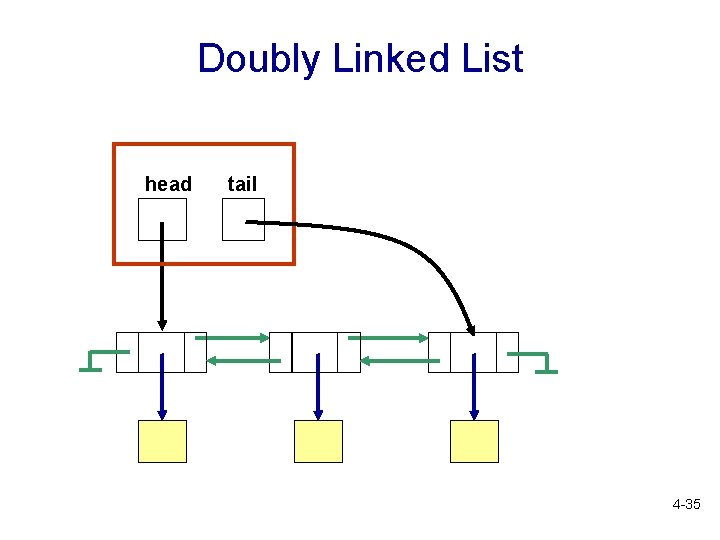• Slides: 35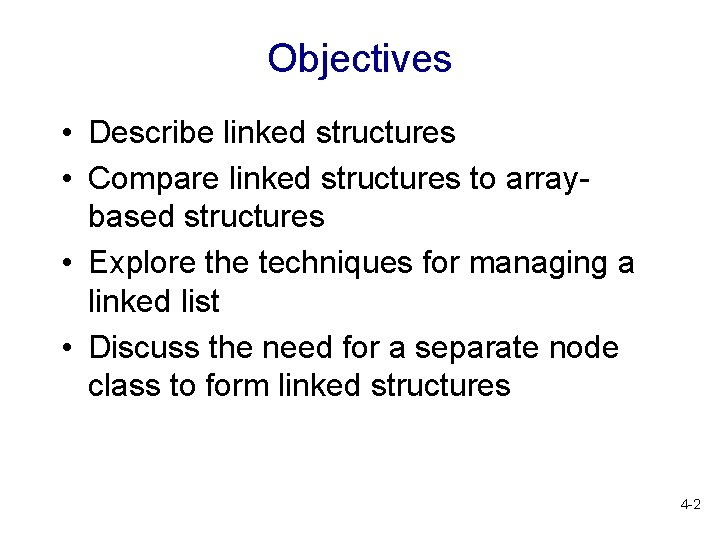Objectives • Describe linked structures • Compare linked structures to arraybased structures • Explore the techniques for managing a linked list • Discuss the need for a separate node class to form linked structures 4 -2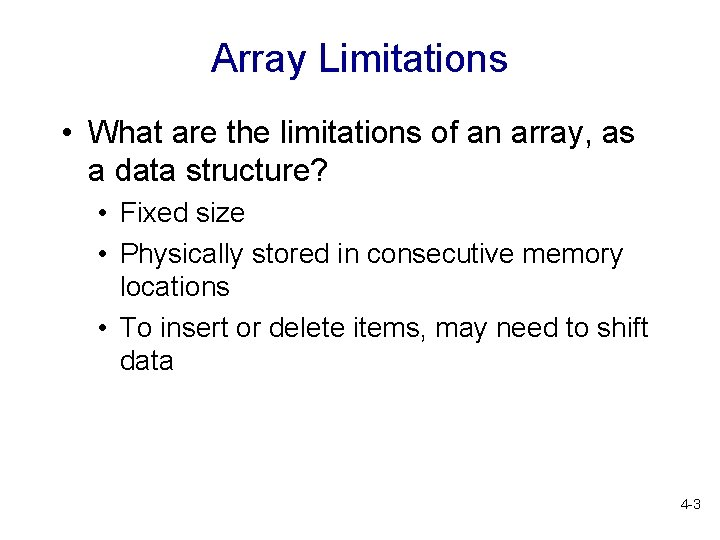Array Limitations • What are the limitations of an array, as a data structure? • Fixed size • Physically stored in consecutive memory locations • To insert or delete items, may need to shift data 4 -3Linked Data Structures • A linked data structure consists of items that are linked to other items • How? each item points to another item Memory Addr 1 Addr 2 Addr 3 null data 1 data 2 4 -4Linked Data Structures • A linked data structure consists of items that are linked to other items • How? each item points to another item • Singly linked list: each item points to the next item • Doubly linked list: each item points to the next item and to the previous item 4 -5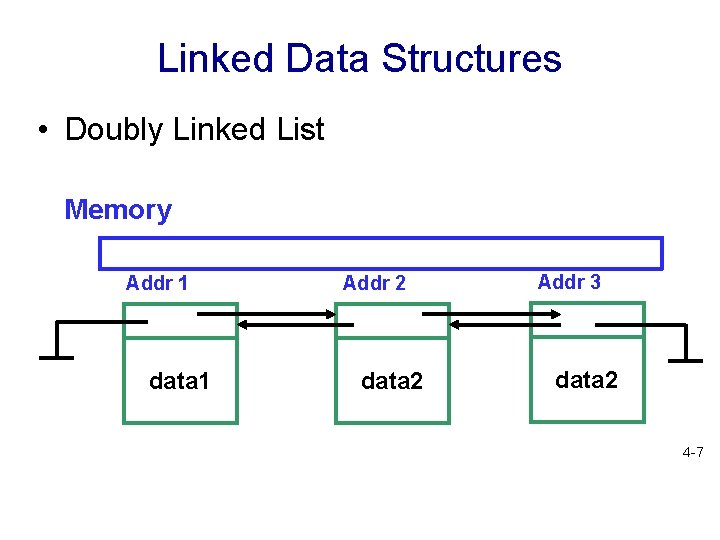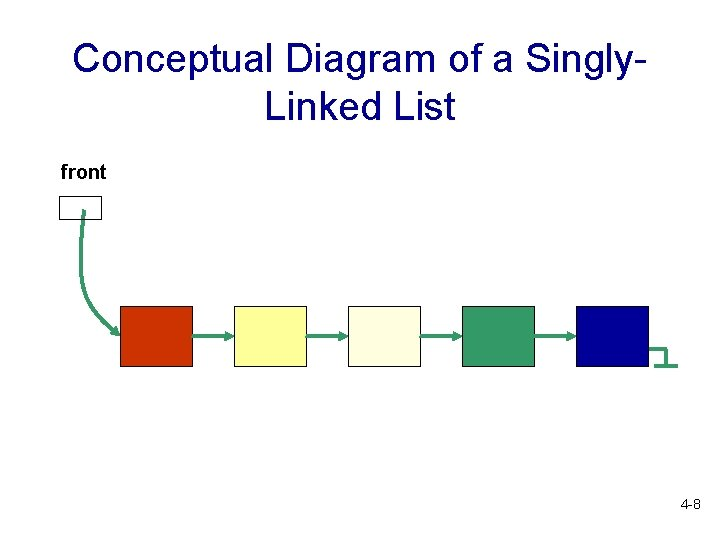Conceptual Diagram of a Singly. Linked List front 4 -8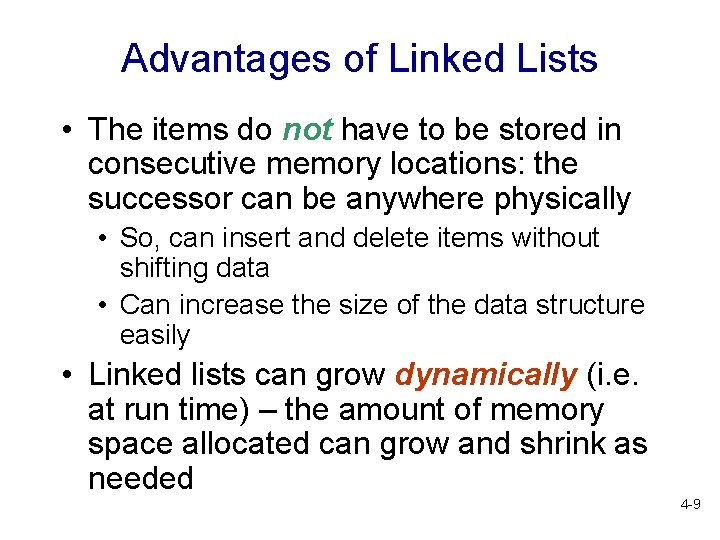Advantages of Linked Lists • The items do not have to be stored in consecutive memory locations: the successor can be anywhere physically • So, can insert and delete items without shifting data • Can increase the size of the data structure easily • Linked lists can grow dynamically (i. e. at run time) – the amount of memory space allocated can grow and shrink as needed 4 -9Nodes • A linked list is an ordered sequence of items called nodes • A node is the basic unit of representation in a linked list • A node in a singly linked list consists of two fields: • A data portion • A link (pointer) to the next node in the structure • The first item (node) in the linked list is accessed via a front or head pointer • The linked list is defined by its head (this is its starting point) 4 -10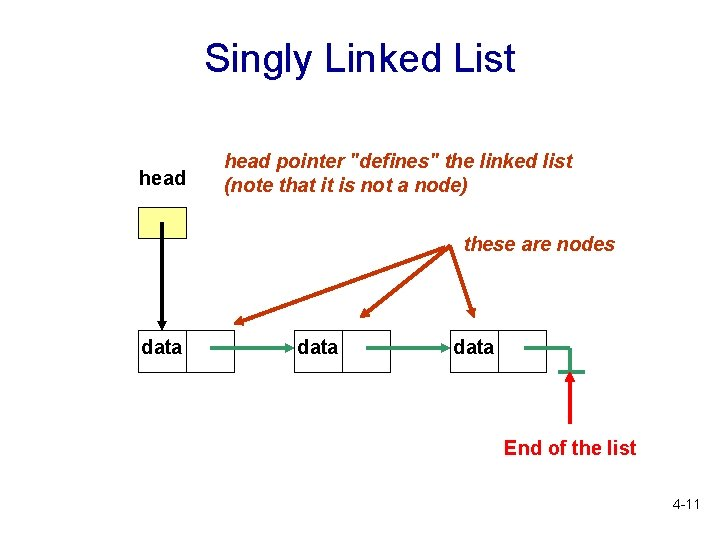Singly Linked List head pointer "defines" the linked list (note that it is not a node) these are nodes data End of the list 4 -11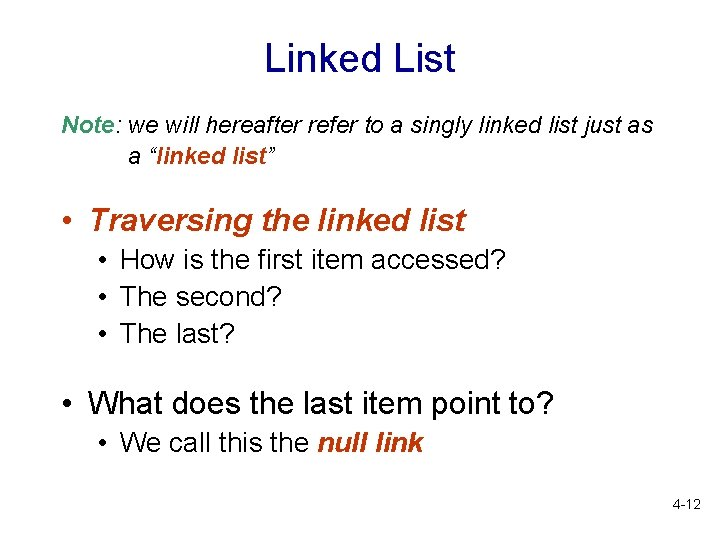Linked List Note: we will hereafter refer to a singly linked list just as a “linked list” • Traversing the linked list • How is the first item accessed? • The second? • The last? • What does the last item point to? • We call this the null link 4 -12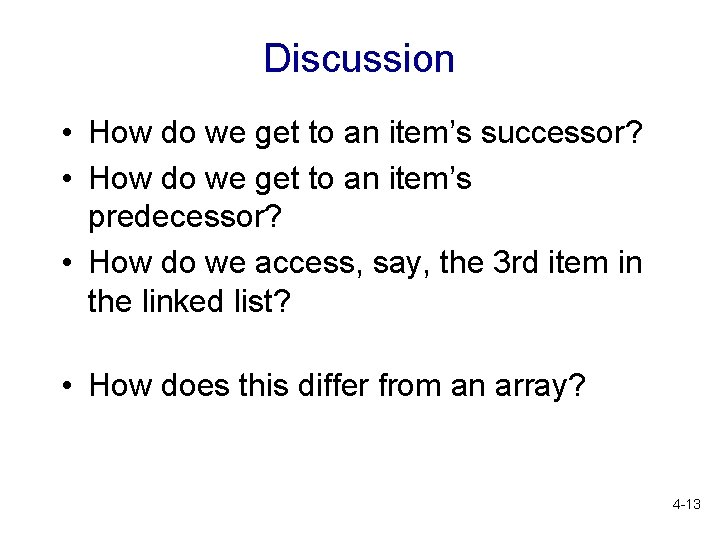Discussion • How do we get to an item’s successor? • How do we get to an item’s predecessor? • How do we access, say, the 3 rd item in the linked list? • How does this differ from an array? 4 -13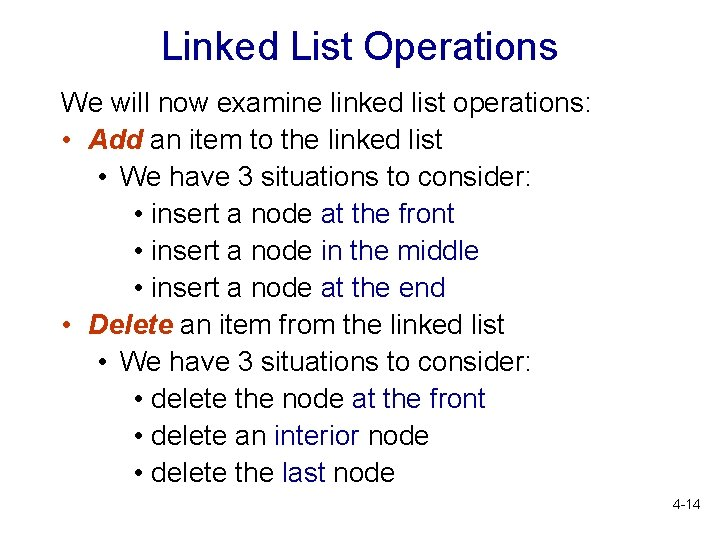Linked List Operations We will now examine linked list operations: • Add an item to the linked list • We have 3 situations to consider: • insert a node at the front • insert a node in the middle • insert a node at the end • Delete an item from the linked list • We have 3 situations to consider: • delete the node at the front • delete an interior node • delete the last node 4 -14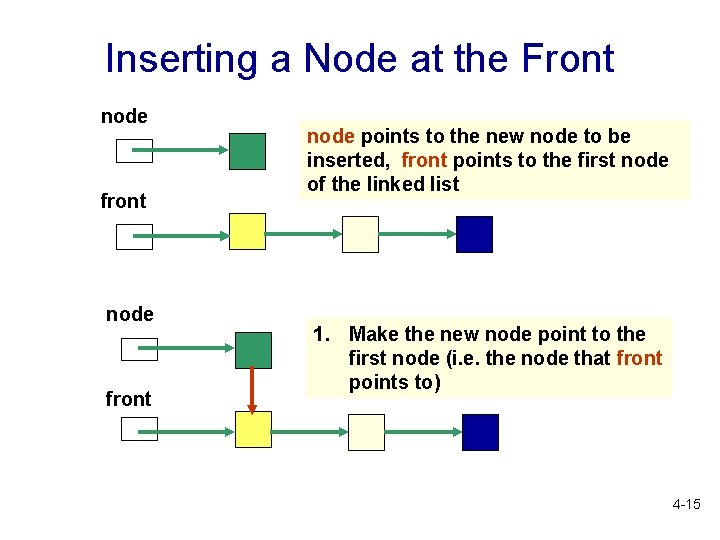Inserting a Node at the Front node front node points to the new node to be inserted, front points to the first node of the linked list 1. Make the new node point to the first node (i. e. the node that front points to) 4 -15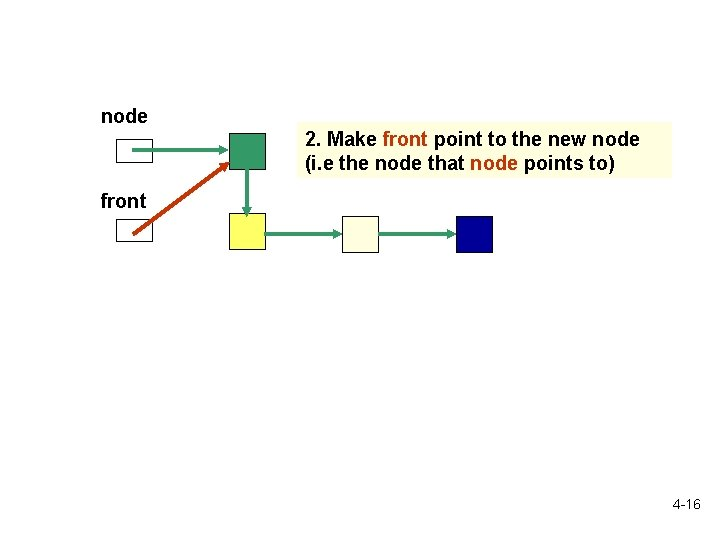node 2. Make front point to the new node (i. e the node that node points to) front 4 -16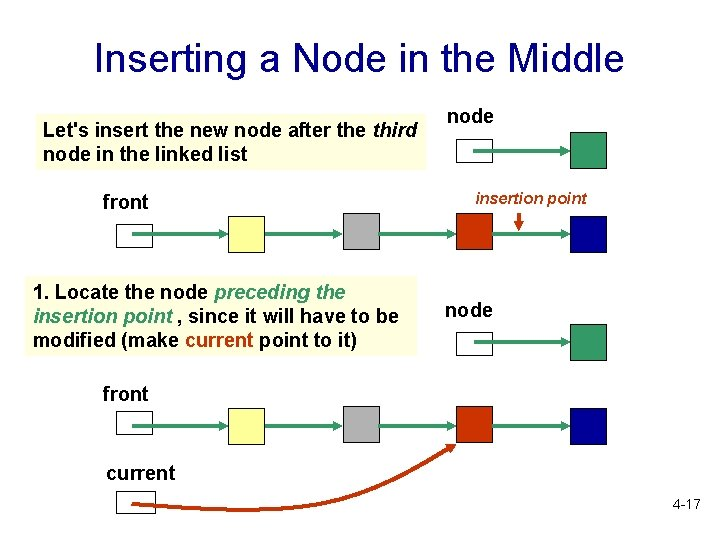Inserting a Node in the Middle Let's insert the new node after the third node in the linked list front 1. Locate the node preceding the insertion point , since it will have to be modified (make current point to it) node insertion point node front current 4 -17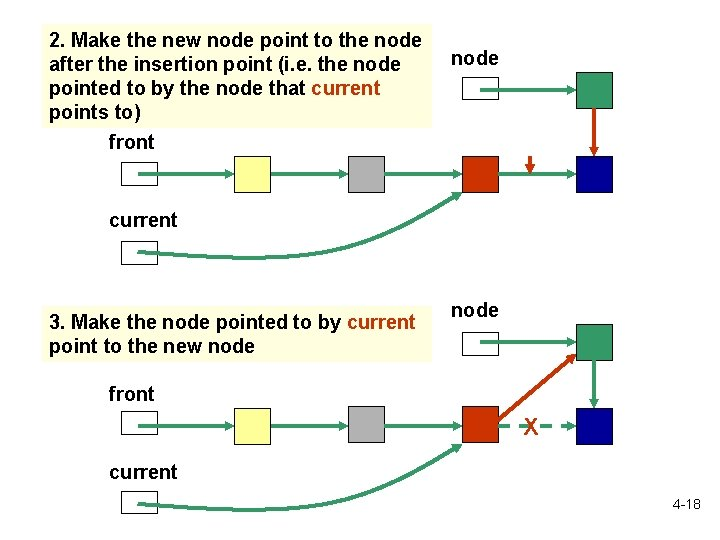2. Make the new node point to the node after the insertion point (i. e. the node pointed to by the node that current points to) node front current 3. Make the node pointed to by current point to the new node front X current 4 -18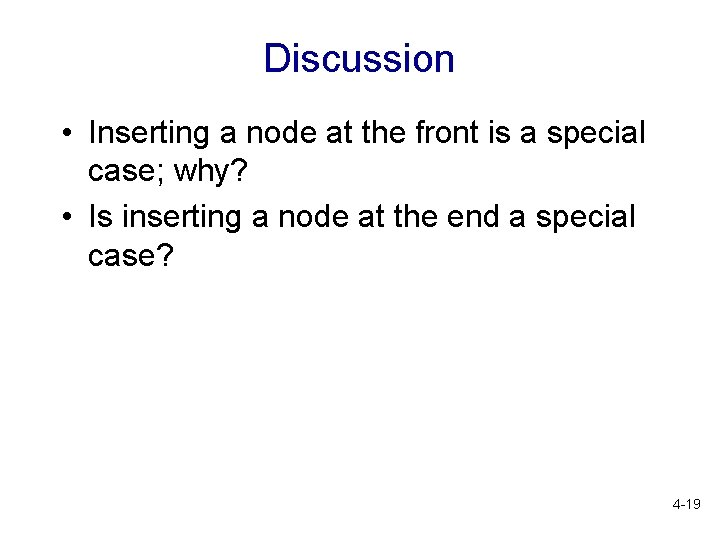Discussion • Inserting a node at the front is a special case; why? • Is inserting a node at the end a special case? 4 -19Deleting the First Node front points to the first node in the linked list, which points to the second node front Make front point to the second node (i. e. the node pointed to by the first node) front X 4 -20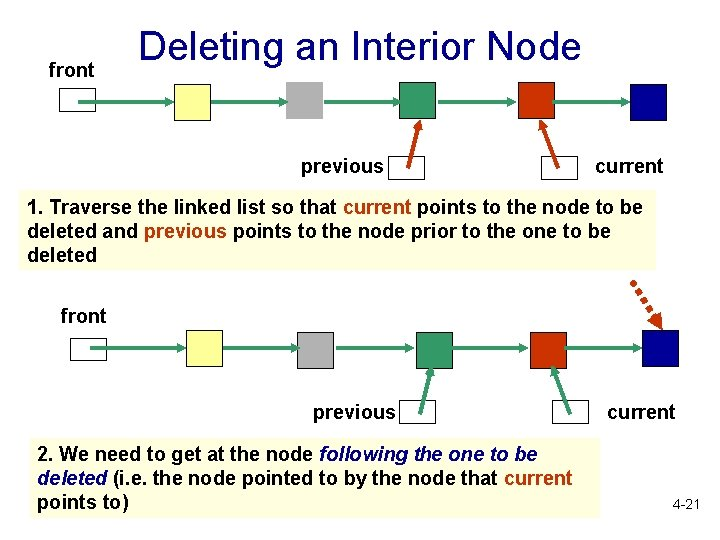front Deleting an Interior Node previous current 1. Traverse the linked list so that current points to the node to be deleted and previous points to the node prior to the one to be deleted front previous 2. We need to get at the node following the one to be deleted (i. e. the node pointed to by the node that current points to) current 4 -21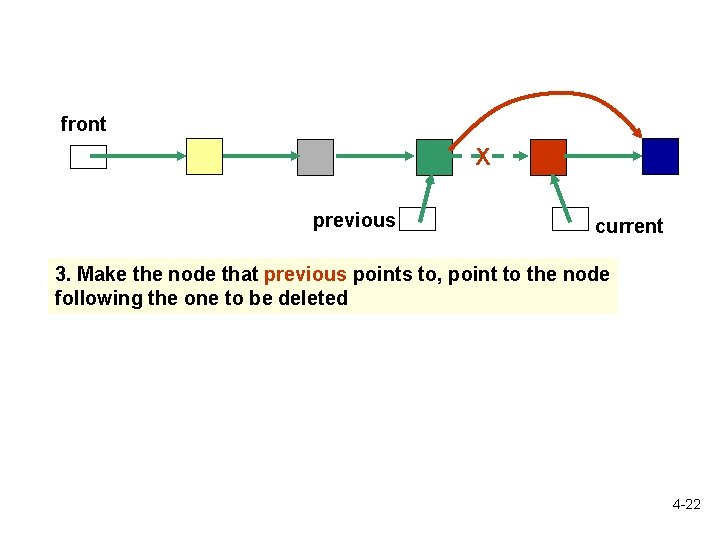front X previous current 3. Make the node that previous points to, point to the node following the one to be deleted 4 -22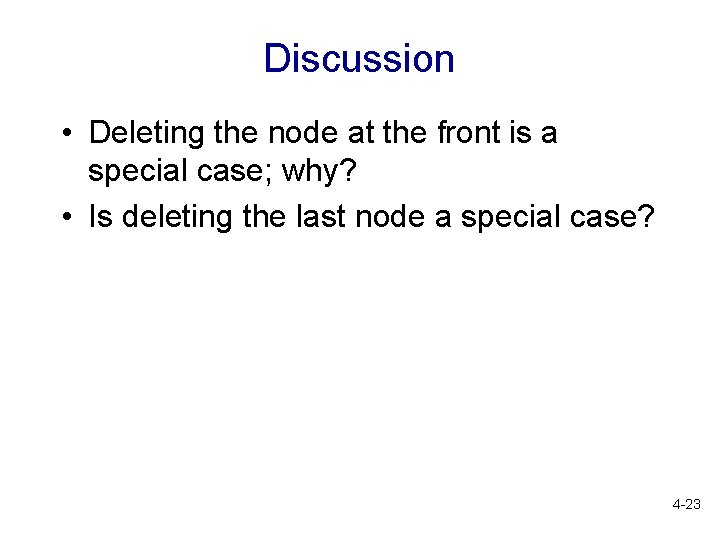Discussion • Deleting the node at the front is a special case; why? • Is deleting the last node a special case? 4 -23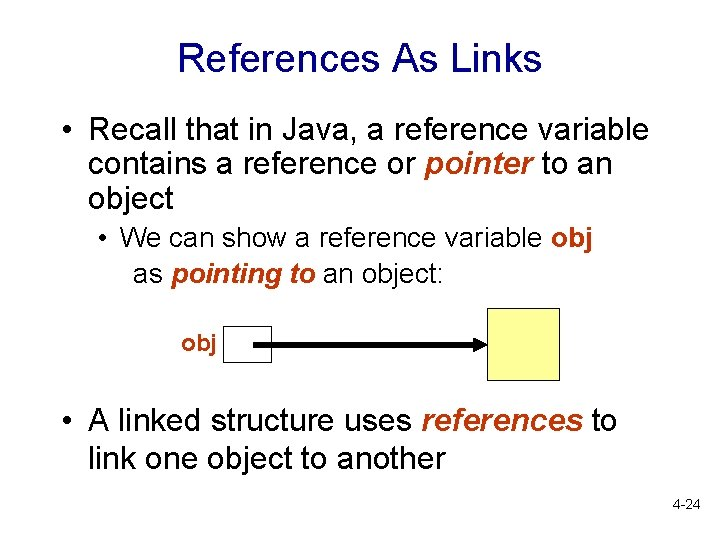References As Links • Recall that in Java, a reference variable contains a reference or pointer to an object • We can show a reference variable obj as pointing to an object: obj • A linked structure uses references to link one object to another 4 -24Implementation of Linked List • In Java, a linked list is a list of node objects, each of which consists of two references: • A reference to the data object • A reference to the next node object • The head pointer is the reference to the linked list, i. e. to the first node object in the linked list • The last node has the null value as its reference to the “next” node object 4 -25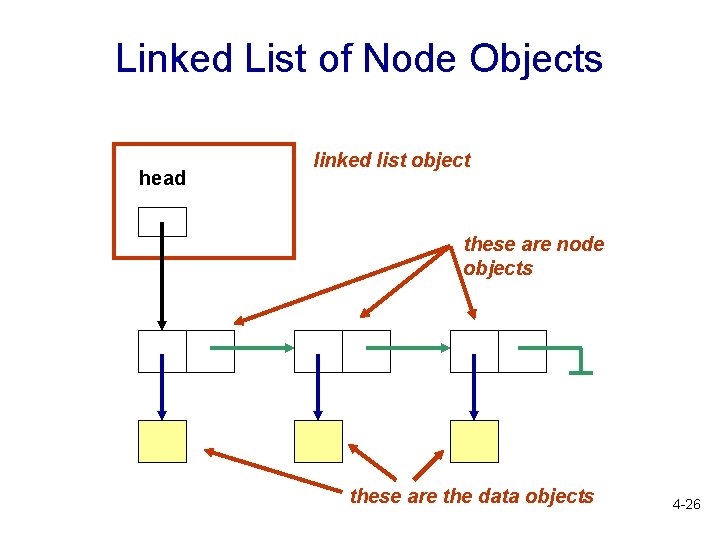Linked List of Node Objects head linked list object these are node objects these are the data objects 4 -26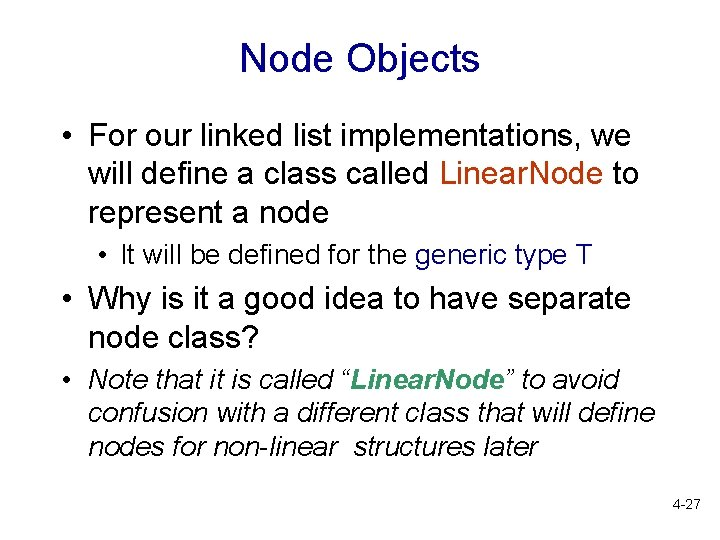Node Objects • For our linked list implementations, we will define a class called Linear. Node to represent a node • It will be defined for the generic type T • Why is it a good idea to have separate node class? • Note that it is called “Linear. Node” to avoid confusion with a different class that will define nodes for non-linear structures later 4 -27The Linear. Node Class • Attributes (instance variables): • element: a reference to the data object • next : a reference to the next node • so it will be of type Linear. Node element next data object 4 -28The Linear. Node Class • Methods: we only need • Getters • Setters 4 -29public class Linear. Node<T> { private Linear. Node<T> next; private T element; public Linear. Node( ){ next = null; element = null; } Linear. Node. java public Linear. Node (T elem){ next = null; element = elem; } // cont’d. . 4 -30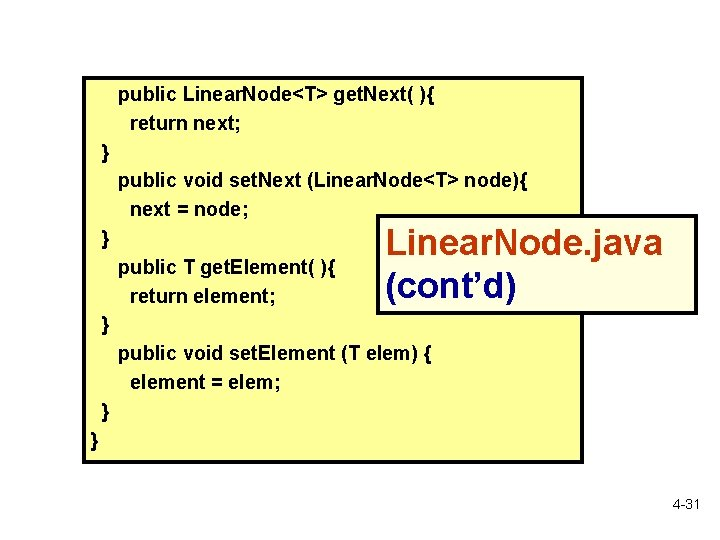public Linear. Node<T> get. Next( ){ return next; } public void set. Next (Linear. Node<T> node){ next = node; } public T get. Element( ){ return element; Linear. Node. java (cont’d) } public void set. Element (T elem) { element = elem; } } 4 -31Example: Create a Linear. Node Object • Example: create a node that contains the integer 7 Integer int. Obj = new Integer(7); Linear. Node<Integer> inode = new Linear. Node<Integer> (int. Obj); or Linear. Node<Integer> inode = new Linear. Node<Integer> (new Integer(7)); 4 -32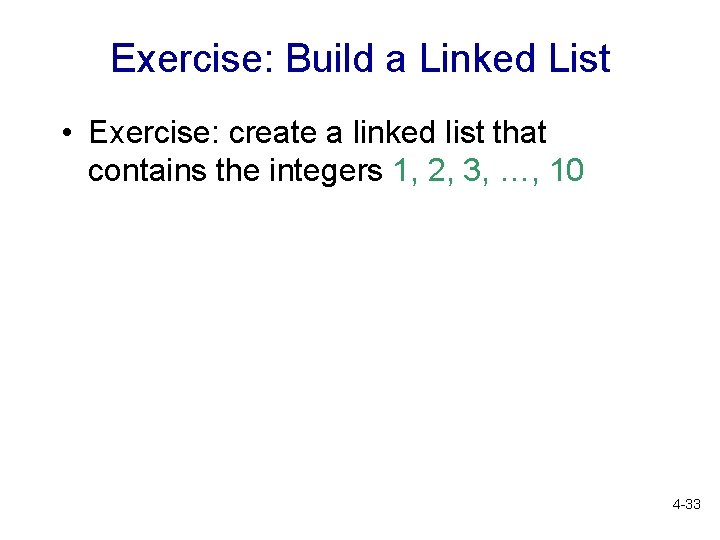Exercise: Build a Linked List • Exercise: create a linked list that contains the integers 1, 2, 3, …, 10 4 -33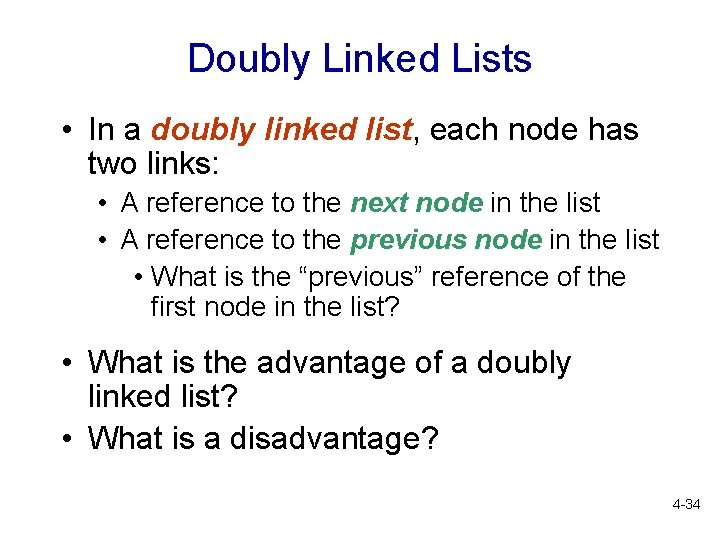Doubly Linked Lists • In a doubly linked list, each node has two links: • A reference to the next node in the list • A reference to the previous node in the list • What is the “previous” reference of the first node in the list? • What is the advantage of a doubly linked list? • What is a disadvantage? 4 -34